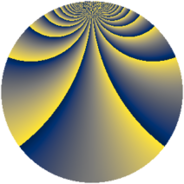# Properties

 Label 1470.2.boLevel $1470$ Weight $2$ Character orbit 1470.bo Rep. character $\chi_{1470}(101,\cdot)$ Character field $\Q(\zeta_{42})$ Dimension $912$ Sturm bound $672$

# Related objects

## Defining parameters

 Level: $$N$$ $$=$$ $$1470 = 2 \cdot 3 \cdot 5 \cdot 7^{2}$$ Weight: $$k$$ $$=$$ $$2$$ Character orbit: $$[\chi]$$ $$=$$ 1470.bo (of order $$42$$ and degree $$12$$) Character conductor: $$\operatorname{cond}(\chi)$$ $$=$$ $$147$$ Character field: $$\Q(\zeta_{42})$$ Sturm bound: $$672$$

## Dimensions

The following table gives the dimensions of various subspaces of $$M_{2}(1470, [\chi])$$.

Total New Old
Modular forms 4128 912 3216
Cusp forms 3936 912 3024
Eisenstein series 192 0 192

## Trace form

 $$912q - 76q^{4} - 14q^{6} - 16q^{7} - 16q^{9} + O(q^{10})$$ $$912q - 76q^{4} - 14q^{6} - 16q^{7} - 16q^{9} + 76q^{16} + 8q^{18} + 14q^{21} + 6q^{24} + 76q^{25} + 84q^{27} - 8q^{28} + 2q^{30} - 24q^{31} + 24q^{33} + 10q^{36} + 72q^{37} + 12q^{39} + 80q^{42} - 16q^{43} - 6q^{45} + 156q^{46} + 140q^{49} + 12q^{51} - 32q^{52} + 36q^{54} + 40q^{57} + 104q^{58} - 164q^{61} - 16q^{63} + 152q^{64} + 48q^{66} + 16q^{67} + 56q^{69} - 12q^{70} - 8q^{72} - 32q^{78} - 16q^{79} + 120q^{81} - 8q^{84} + 152q^{87} + 56q^{91} - 4q^{93} + 64q^{94} + 6q^{96} - 256q^{99} + O(q^{100})$$

## Decomposition of $$S_{2}^{\mathrm{new}}(1470, [\chi])$$ into newform subspaces

The newforms in this space have not yet been added to the LMFDB.

## Decomposition of $$S_{2}^{\mathrm{old}}(1470, [\chi])$$ into lower level spaces

$$S_{2}^{\mathrm{old}}(1470, [\chi]) \cong$$ $$S_{2}^{\mathrm{new}}(147, [\chi])$$$$^{\oplus 4}$$$$\oplus$$$$S_{2}^{\mathrm{new}}(294, [\chi])$$$$^{\oplus 2}$$$$\oplus$$$$S_{2}^{\mathrm{new}}(735, [\chi])$$$$^{\oplus 2}$$